# 跟我一起学opencv 第二课之图像的掩膜操作

### 2.掩膜的作用

• 提取感兴趣区，用预先制作的感兴趣区掩模与待处理图像相乘，得到感兴趣区图像，感兴趣区内图像值保持不变，而区外图像值都为0。
• 屏蔽作用，用掩模对图像上某些区域作屏蔽，使其不参加处理或不参加处理参数的计算，或仅对屏蔽区作处理或统计。
• 结构特征提取，用相似性变量或图像匹配方法检测和提取图像中与掩模相似的结构特征。
• 特殊形状图像的制作
• 掩膜是一种图像滤镜的模板，实用掩膜经常处理的是遥感图像。当提取道路或者河流，或者房屋时，通过一个N * N的矩阵来对图像进行像素过滤，然后将我们需要的地物或者标志突出显示出来。这个矩阵就是一种掩膜。
• 在OpenCV的中，掩模操作是相对简单的。大致的意思是，通过一个掩模矩阵，重新计算图像中的每一个像素值。掩模矩阵控制了旧图像当前位置以及周围位置像素对新图像当前位置像素值的影响力度。用数学术语讲，即我们自定义一个权重表

### 3.通过掩膜操作实现图像对比图的改变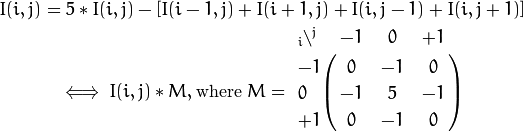```Mat kern = (Mat_<char>(3,3) <<  0, -1,  0,
-1,  5, -1,
0, -1,  0);```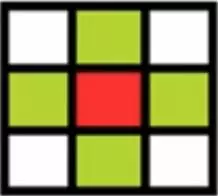### 4.像素范围处理saturate_cast <typename _Tp>（）

• saturate_cast <UCHAR>（ - 100），返回0
• saturate_cast <UCHAR>（288），返回255
• saturate_cast <UCHAR>（100），返回100

### 5.自定义使用掩膜操作实现图像对比度的提高

（1）获取对象像素指针

------CV_Assert(myImage.depth())

------Mat.ptr<uchar>(int i=0)获取像素矩阵的指针，索引i表示第几行，从0行开始计数

------获得当前行指针const uchar * current= myImage.ptr<uchar>(row);

------获取当前像素点P(row,col)的像素值p(row,col)=current[col]

```using namespace std;
using namespace cv;

int main(int argc, char **argv)
{
if (src.empty())
{
cout << "can not load imagefile...." << endl;
return -1;
}
namedWindow("in image win", CV_WINDOW_AUTOSIZE);
imshow("in image win", src);

Mat dst;
dst = Mat::zeros(src.size(), src.type());//用原图像的尺寸和类型初始化目标图像并将所有像素值清零
int cols = src.cols * src.channels();//图像矩阵的宽度(列数)=原图像列数*图像的通道数,因为掩膜矩阵要在图像矩阵内，所以计算时要给列和行减1
int rows = src.rows;//图像的高度(行数)
int offsetx = src.channels();//掩膜计算偏移量等于图像通道数
for (int row = 1; row < (rows - 1); row++)//从第二行开始到倒数第二行结束
{
const uchar* previous = src.ptr<uchar>(row - 1);//上一行
const uchar* current = src.ptr<uchar>(row);//获取row行的像素指针
const uchar* next = src.ptr<uchar>(row + 1);//下一行
uchar* outcurrent = dst.ptr<uchar>(row);//输出图像的当前行像素指针
for (int col = offsetx; col < (cols - offsetx); col++)//从第二列(按通道偏移计算)到倒数第二列
{
outcurrent[col] = saturate_cast<uchar>( 5 * current[col] - (current[col - offsetx] + current[col + offsetx] + previous[col] + next[col]));//分别对应中间，左右，右边，上面，下面；使用掩膜修改输出图像每一点的像素值,然后saturate_cast限制像素范围为0-255
}

}
//将修改完的输出图像在窗口展示出来
namedWindow("out win", CV_WINDOW_AUTOSIZE);
imshow("out win", dst);

waitKey(0);
return 0;
}```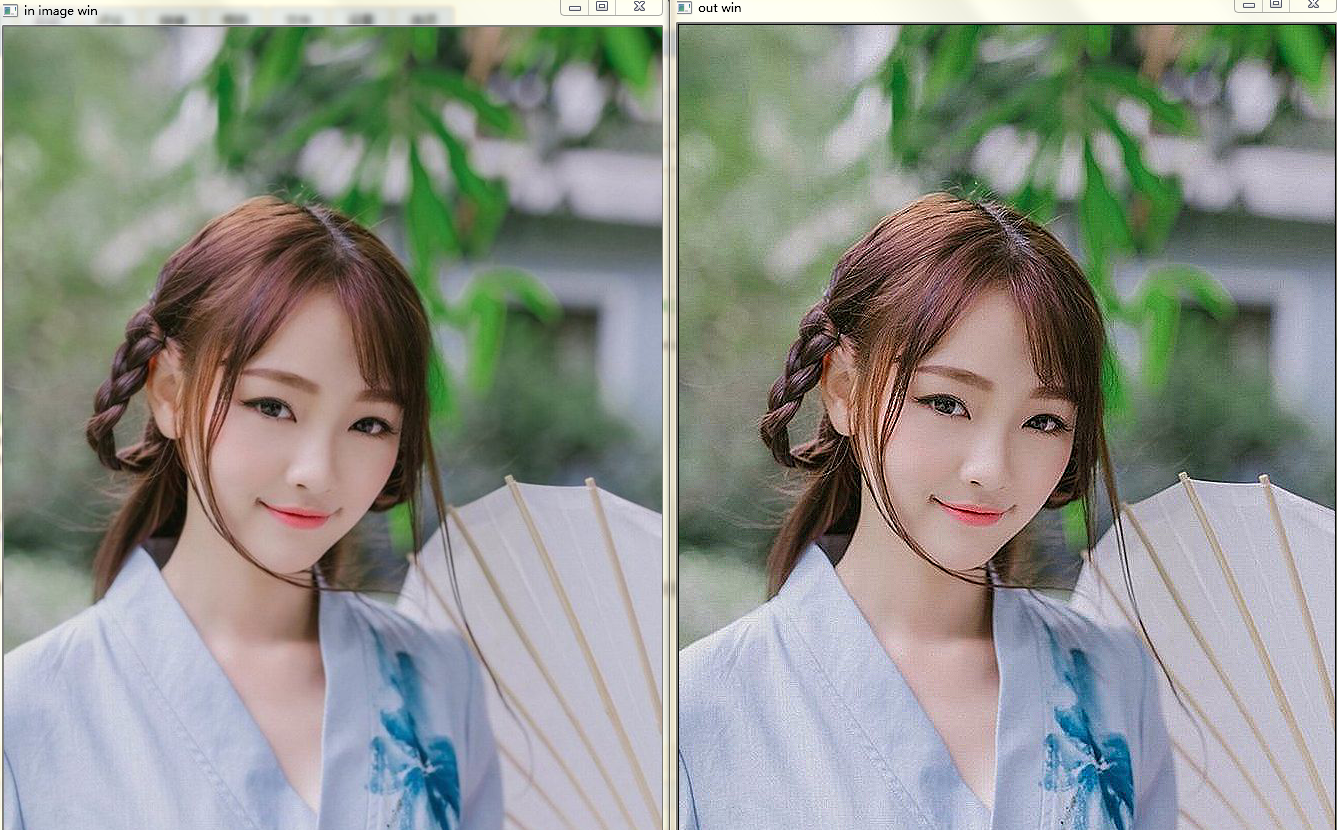### 6.使用opencv 的API实现图像掩膜

1.定义一个3*3的掩膜如下

`Mat kernal = (Mat_<char>(3, 3) << 0, -1, 0, -1, 5, -1, 0, -1, 0);`

2.使用opencv函数进行掩膜操作

`    filter2D(src, dst, src.depth(), kernal);`

```#include<opencv2\opencv.hpp>
#include<iostream>

using namespace std;
using namespace cv;

int main(int argc, char **argv)
{
if (src.empty())
{
cout << "can not load imagefile...." << endl;
return -1;
}
namedWindow("in image win", CV_WINDOW_AUTOSIZE);
imshow("in image win", src);

Mat dst;
//dst = Mat::zeros(src.size(), src.type());//用原图像的尺寸和类型初始化目标图像并将所有像素值清零
//int cols = src.cols * src.channels();//图像矩阵的宽度(列数)=原图像列数*图像的通道数,因为掩膜矩阵要在图像矩阵内，所以计算时要给列和行减1
//int rows = src.rows;//图像的高度(行数)
//int offsetx = src.channels();//掩膜计算偏移量等于图像通道数
//for (int row = 1; row < (rows - 1); row++)//从第二行开始到倒数第二行结束
//{
//    const uchar* previous = src.ptr<uchar>(row - 1);//上一行
//    const uchar* current = src.ptr<uchar>(row);//获取row行的像素指针
//    const uchar* next = src.ptr<uchar>(row + 1);//下一行
//    uchar* outcurrent = dst.ptr<uchar>(row);//输出图像的当前行像素指针
//    for (int col = offsetx; col < (cols - offsetx); col++)//从第二列(按通道偏移计算)到倒数第二列
//    {
//        outcurrent[col] = saturate_cast<uchar>( 5 * current[col] - (current[col - offsetx] + current[col + offsetx] + previous[col] + next[col]));//分别对应中间，左右，右边，上面，下面；使用掩膜修改输出图像每一点的像素值,然后saturate_cast限制像素范围为0-255
//    }

//}

//创建掩膜 3*3
Mat kernal = (Mat_<char>(3, 3) << 0, -1, 0, -1, 5, -1, 0, -1, 0);
//掩膜操作
filter2D(src, dst, src.depth(), kernal);

//将修改完的输出图像在窗口展示出来
namedWindow("dst win", CV_WINDOW_AUTOSIZE);
imshow("dst win", dst);

waitKey(0);
return 0;
}```posted on 2019-04-19 17:49 lovejobs 阅读( ...) 评论( ...) 编辑 收藏

##### 热门推荐
•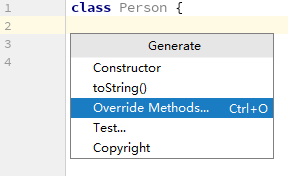浏览(655)
•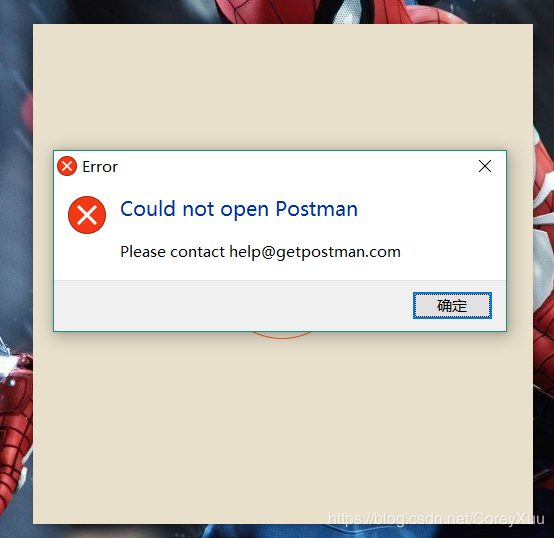浏览(565)
•浏览(548)
•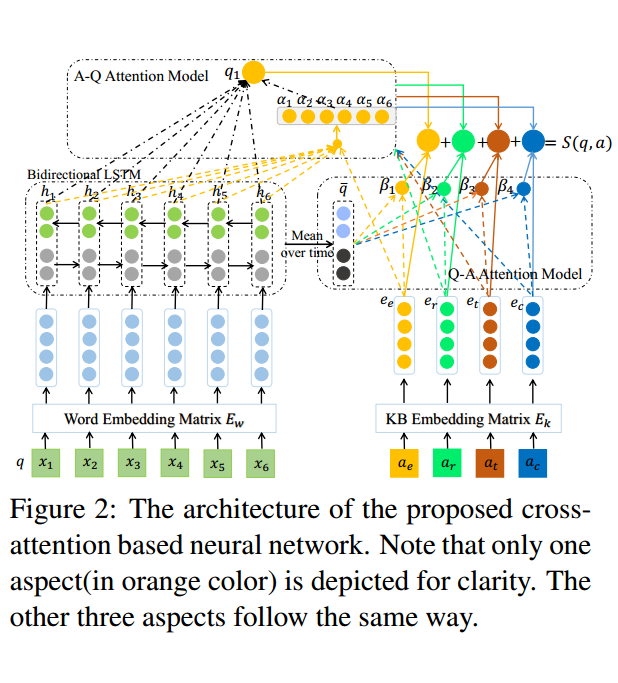浏览(488)
•浏览(434)
•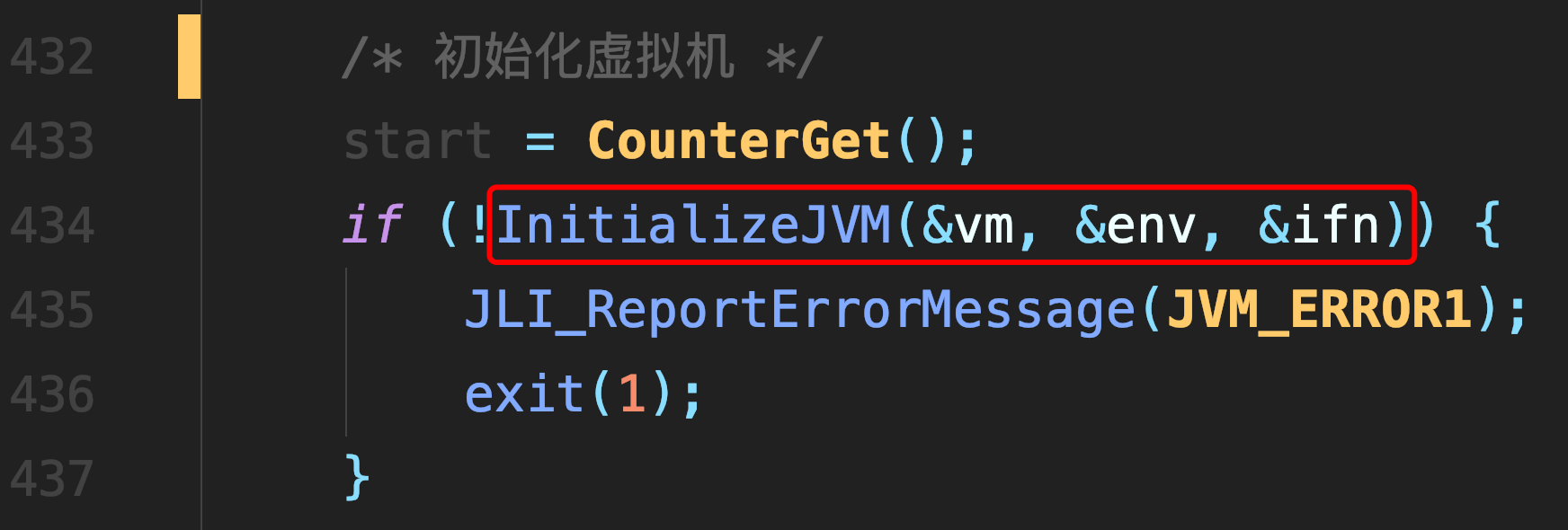浏览(424)
•浏览(381)
•浏览(367)
•浏览(362)
•浏览(358)